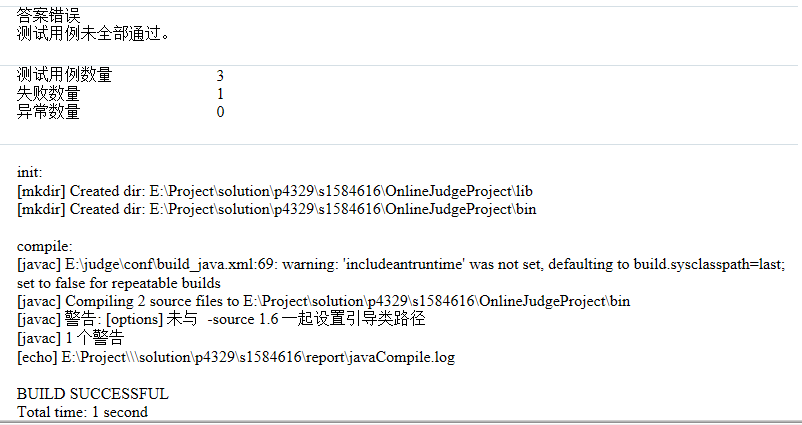Public static boolean isAutoMorphicNum( int num)

num 需要判断的数

true 是自守数
false 不是自守数

JAVA语言基础：数字处理

public static boolean isAutoMorphicNum(int num)
{
String input = num + "";
String power = num * num + "";
System.out.println(num * num);
if (input.equals(power.substring(power.length() - input.length()))) {
return true;
}
return false;
}5个回答

String input = num.toString();
String power = (num * num).toString(); 呢#include
#include
int main()
{
long mul,number,k,ll,kk;
for (number = 0;number {
//由number的位数确定截取数字，进行乘法时的系数k
for(mul = number,k = 1;(mul /= 10)>0;k*=10);

``````    kk = k*10;  //截取部分乘积时的系数
mul = 0;    //积的最后N位
ll = 10;    //截取乘数时的系数
while(k>0)
{
//(部分积+截取被乘数的后N位*截取乘数的第M位)%kk再截取部分积
mul = (mul+(number%(k*10))*(number%ll-number%(ll/10)))%kk;
k/=10;  //截取被乘数时的系数
ll*=10;
}
if (number == mul)
{
printf("%ld  ",number);
}
}

return 0;
``````

}

``````
``````